Home
Hostname: page-component-55597f9d44-ssw5r Total loading time: 0.3 Render date: 2022-08-10T09:13:47.616Z Has data issue: true Feature Flags: { "shouldUseShareProductTool": true, "shouldUseHypothesis": true, "isUnsiloEnabled": true, "useRatesEcommerce": false, "useNewApi": true } hasContentIssue true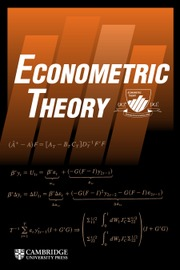Econometric Theory

# COINTEGRATION IN FUNCTIONAL AUTOREGRESSIVE PROCESSES

Published online by Cambridge University Press:  22 November 2019

*
Address correspondence to Paolo Paruolo, European Commission, Joint Research Centre, Via E. Fermi 2749, I-21027 Ispra (VA), Italy; e-mail: paolo.paruolo@ec.europa.eu.

## Abstract

This article defines the class of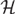${\cal H}$-valued autoregressive (AR) processes with a unit root of finite type, where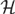${\cal H}$ is a possibly infinite-dimensional separable Hilbert space, and derives a generalization of the Granger–Johansen Representation Theorem valid for any integration order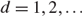$d = 1,2, \ldots$. An existence theorem shows that the solution of an AR process with a unit root of finite type is necessarily integrated of some finite integer order d, displays a common trends representation with a finite number of common stochastic trends, and it possesses an infinite-dimensional cointegrating space when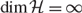${\rm{dim}}{\cal H} = \infty$. A characterization theorem clarifies the connections between the structure of the AR operators and (i) the order of integration, (ii) the structure of the attractor space and the cointegrating space, (iii) the expression of the cointegrating relations, and (iv) the triangular representation of the process. Except for the fact that the dimension of the cointegrating space is infinite when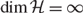${\rm{dim}}{\cal H} = \infty$, the representation of AR processes with a unit root of finite type coincides with the one of finite-dimensional VARs, which can be obtained setting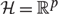${\cal H} = ^p$ in the present results.

Type
ARTICLES
Information
Econometric Theory , October 2020 , pp. 803 - 839

## Access options

Get access to the full version of this content by using one of the access options below. (Log in options will check for institutional or personal access. Content may require purchase if you do not have access.)

## Footnotes

The article benefited from useful comments from the Editor, Peter C.B. Phillips, three anonymous referees and conference and seminar participants at 2018 NBER-NSF Time Series Conference, University of California San Diego, University of Bologna and ICEEE 2019, University of Lecce. The idea of the present article was conceived while the first author was visiting the Department of Economics, Indiana University, in January 2017; the hospitality of Yoosoon Chang and Joon Park is gratefully acknowledged. During the revision of the article in September 2018, the first author visited the Department of Economics, University of California San Diego, and the hospitality of Brendan K. Beare is gratefully acknowledged. The first author acknowledges partial financial support from MIUR PRIN grant 2010J3LZEN. The views expressed in this article do not necessarily reflect those of the authors’ institutions.

## References

Beare, B. (2017) The Chang-Kim-Park model of cointegrated density-valued time series cannot accommodate a stochastic trend. Econ Journal Watch 14, 133137.Google Scholar
Beare, B., Seo, J., & Seo, W. (2017) Cointegrated linear processes in Hilbert space. Journal of Time Series Analysis 38, 10101027.CrossRefGoogle Scholar
Beare, B. & Seo, W. (2019) Representation of I(1) and I(2) autoregressive Hilbertian processes. Econometric Theory, https://doi.org/10.1017/S0266466619000276.Google Scholar
Ben-Israel, A. & Greville, T. (2003) Generalized Inverses: Theory and Applications, 2nd ed. Springer.Google Scholar
Bosq, D. (2000) Linear Processes in Function Spaces. Springer-Verlag.CrossRefGoogle Scholar
Bourguignon, F., Ferreira, F.H., & Lustig, N. (2005) The Microeconomics of Income Distribution Dynamics in East Asia and Latin America. World Bank and Oxford University PressGoogle Scholar
Chang, Y., Hu, B., & Park, J. (2016a). On the Error Correction Model for Functional Time Series with Unit Roots. Mimeo, Indiana University.Google Scholar
Chang, Y., Kim, C., & Park, J. (2016b) Nonstationarity in time series of state densities. Journal of Econometrics 192, 152167.CrossRefGoogle Scholar
Cochrane, J.H. & Piazzesi, M. (2005) Bond risk premia. American Economic Review 95(1), 138160.CrossRefGoogle Scholar
Franchi, M. & Paruolo, P. (2016) Inverting a matrix function around a singularity via local rank factorization. SIAM Journal of Matrix Analysis and Applications 37, 774797.CrossRefGoogle Scholar
Franchi, M. & Paruolo, P. (2019) A general inversion theorem for cointegration. Econometric Reviews 38, 11761201.CrossRefGoogle Scholar
Gabrys, R., Hörmann, S., & Kokoszka, P. (2013) Monitoring the intraday volatility pattern. Journal of Time Series Econometrics 5, 87116.CrossRefGoogle Scholar
Gohberg, I., Goldberg, S., & Kaashoek, M. (1990) Classes of Linear Operators, vol. 1. Operator Theory. Birkhäuser Verlag.CrossRefGoogle Scholar
Gohberg, I., Goldberg, S., & Kaashoek, M. (2003) Basic Classes of Linear Operators. Birkhäuser.CrossRefGoogle Scholar
Granger, C.W.J. & Lee, T.H. (1989) Investigation of production, sales and inventory relationships using multicointegration and non-symmetric error correction models. Journal of Applied Econometrics 4, S145S159.CrossRefGoogle Scholar
Gregoir, S. (1999) Multivariate time series with various hidden unit roots, Part I. Econometric Theory 15, 435468.CrossRefGoogle Scholar
Hörmann, S., Horváth, L., & Reeder, R. (2013) A functional version of the ARCH model. Econometric Theory 29, 267288.CrossRefGoogle Scholar
Hörmann, S. & Kokoszka, P. (2012) Functional time series. Handbook of Statistics 30, 157186.CrossRefGoogle Scholar
Horn, R. & Johnson, C. (2013) Matrix Analysis. Cambridge University Press.Google Scholar
Horváth, L. & Kokoszka, P. (2012) Inference for Functional Data with Application. Springer.CrossRefGoogle Scholar
Howlett, P., Avrachenkov, K., Pearce, C., & Ejov, V. (2009) Inversion of analytically perturbed linear operators that are singular at the origin. Journal of Mathematical Analysis and Applications 353, 6884.CrossRefGoogle Scholar
Hu, B. & Park, J. (2016). Econometric Analysis of Functional Dynamics in the Presence of Persistence. Mimeo, Indiana University.Google Scholar
Johansen, S. (1996) Likelihood-based Inference in Cointegrated Vector Auto-Regressive Models. Oxford University Press.Google Scholar
Kargin, V. & Onatski, A. (2008) Curve forecasting by functional autoregression. Journal of Multivariate Analysis 99, 25082526.CrossRefGoogle Scholar
Kokoszka, P. & Reimherr, M. (2017) Introduction to Functional Data Analysis. Chapman and Hall.CrossRefGoogle Scholar
Petersen, A. & Müller, H.-G. (2016) Functional data analysis for density functions by transformation to a Hilbert space. Annals of Statistics 44(1), 183218.CrossRefGoogle Scholar
Phillips, P. (1991a) Optimal inference in cointegrated systems. Econometrica 59, 283306.CrossRefGoogle Scholar
Phillips, P. (1991b). Spectral regression for cointegrated time series. In Barnett, W. (ed.), Nonparametric and Semiparametric Methods in Economics and Statistics, pp. 413435. Cambridge University Press.Google Scholar
Piketty, T. (2014) Capital in the Twenty-First Century. The Belknap Press of Harvard University Press.CrossRefGoogle ScholarPubMed
Seo, W. & Beare, B. (2019) Cointegrated linear processes in Bayes Hilbert space. Statistics and Probability Letters 147, 9095.CrossRefGoogle Scholar
Stock, J. & Watson, M. (1993) A simple estimator of cointegrating vectors in higher order integrated systems. Econometrica 61, 783820.CrossRefGoogle Scholar
6
Cited by

# Save article to Kindle

Note you can select to save to either the @free.kindle.com or @kindle.com variations. ‘@free.kindle.com’ emails are free but can only be saved to your device when it is connected to wi-fi. ‘@kindle.com’ emails can be delivered even when you are not connected to wi-fi, but note that service fees apply.

Find out more about the Kindle Personal Document Service.

COINTEGRATION IN FUNCTIONAL AUTOREGRESSIVE PROCESSES
Available formats
×

# Save article to Dropbox

To save this article to your Dropbox account, please select one or more formats and confirm that you agree to abide by our usage policies. If this is the first time you used this feature, you will be asked to authorise Cambridge Core to connect with your Dropbox account. Find out more about saving content to Dropbox.

COINTEGRATION IN FUNCTIONAL AUTOREGRESSIVE PROCESSES
Available formats
×

# Save article to Google Drive

To save this article to your Google Drive account, please select one or more formats and confirm that you agree to abide by our usage policies. If this is the first time you used this feature, you will be asked to authorise Cambridge Core to connect with your Google Drive account. Find out more about saving content to Google Drive.

COINTEGRATION IN FUNCTIONAL AUTOREGRESSIVE PROCESSES
Available formats
×
×